Products of the following reaction

CH3C$\equiv$C.CH2CH3  ... are

(a) CH3CHO + CH3CH2CHO

(b) CH3COOH + CH3COCH3

(c) CH3COOH + HOOC.CH2CH3

(d) CH3COOH + CO2

Concept Questions :-

Preparation & properties of Aldehydes and Ketones
High Yielding Test Series + Question Bank - NEET 2020

Difficulty Level:

Which alkene on ozonolysis gives CH3CH2CHO and CH3COCH3?

(a) CH3CH2CH=C${<}_{{\mathrm{CH}}_{3}}^{{\mathrm{CH}}_{3}}$

(b) CH3CH2CH=CHCH2CH3

(c) CH3CH2CH=CHCH3

(d)

Concept Questions :-

Preparation & properties of Aldehydes and Ketones
High Yielding Test Series + Question Bank - NEET 2020

Difficulty Level:

The major organic product formed from the following reaction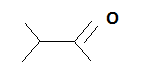... is

1.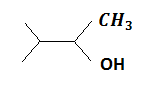2.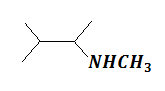3.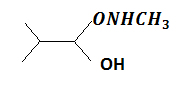4.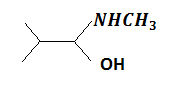Concept Questions :-

Nucleophillic Addition, Reactivity of Alpha Hydrogen in Aldehydes with Uses
High Yielding Test Series + Question Bank - NEET 2020

Difficulty Level:

Benzoic acid gives benzene on being heated with X and phenol gives benzene on being heated with Y. Therefore, X and Y are respectively

(a) sodalime and copper

(b) Zn dust and NaOH

(c) Cu and sodalime

(d) sodalime and zinc dust

Concept Questions :-

Carboxylic Acids
High Yielding Test Series + Question Bank - NEET 2020

Difficulty Level:

Which one of the following on oxidation gives a ketone?

(a) primary alcohol                     (b) secondary alcohol

(c) tertiary alcohol                      (d) All of these

Concept Questions :-

Preparation & properties of Aldehydes and Ketones
High Yielding Test Series + Question Bank - NEET 2020

Difficulty Level:

A carbonyl compound reacts with hydrogen cyanide to form cyanohydrin which on hydrolysis forms a racemic mixture of $\mathrm{\alpha }$-hydroxy acid. The carbonyl compound is

(a) acetaldehyde                              (b) acetone

(c) diethyl ketone                             (d) formaldehyde

Concept Questions :-

Nucleophillic Addition, Reactivity of Alpha Hydrogen in Aldehydes with Uses
High Yielding Test Series + Question Bank - NEET 2020

Difficulty Level:

Consider the following transformations

CH3COOH $\stackrel{{\mathrm{CaCO}}_{3}}{\to }$A$\stackrel{\mathrm{heat}}{\to }$B$\underset{\mathrm{NaOH}}{\overset{{\mathrm{I}}_{2}}{\to }}$C The molecular formula of C is

1. ${\mathrm{CH}}_{3}-\underset{\mathrm{I}}{\overset{\mathrm{OH}}{\underset{|}{\overset{|}{\mathrm{C}}}}}-{\mathrm{CH}}_{3}$

2. ${\mathrm{ICH}}_{2}-{\mathrm{COCH}}_{3}$

3. ${\mathrm{CHI}}_{3}$

4. ${\mathrm{CH}}_{3}\mathrm{I}$

Concept Questions :-

Preparation & properties of Aldehydes and Ketones
High Yielding Test Series + Question Bank - NEET 2020

Difficulty Level:

A and B in the following reactions are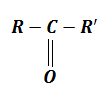$\underset{\mathrm{KCN}}{\overset{\mathrm{HCN}/}{\to }}$A$\stackrel{\mathrm{B}}{\to }$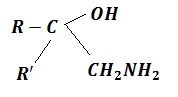(a) A=${\mathrm{RR}}^{\text{'}}{\mathrm{CH}}_{2}\mathrm{CN}$, B=NaOH

(b) A=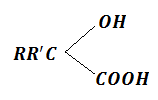, B=${\mathrm{CH}}_{3}$

(c) A=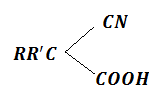, B=${\mathrm{CH}}_{3}$

(d) A=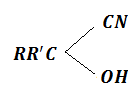, B=${\mathrm{LiAlH}}_{4}$

Concept Questions :-

Nucleophillic Addition, Reactivity of Alpha Hydrogen in Aldehydes with Uses
High Yielding Test Series + Question Bank - NEET 2020

Difficulty Level:

The product formed in aldol condensation is

(a) a beta-hydroxy acid

(b) a beta-hydroxy aldehyde or a beta-hydroxy ketone

(c) an alpha-hydroxy aldehyde or ketone

(d) an alpha, beta unsaturated ester

Concept Questions :-

Preparation & properties of Aldehydes and Ketones
High Yielding Test Series + Question Bank - NEET 2020

Difficulty Level:

Consider the following reaction,

Phenol$\stackrel{\mathrm{Zn}-\mathrm{dust}}{\to }$X$\underset{\mathrm{Anhy}.{\mathrm{AlCl}}_{3}}{\overset{{\mathrm{CH}}_{3}\mathrm{Cl}}{\to }}$Y$\stackrel{\mathrm{Alk}.{\mathrm{KMnO}}_{4}}{\to }$

The product Z is

(a) toluene

(b) benzaldehyde

(c) benzoic acid

(d) benzene